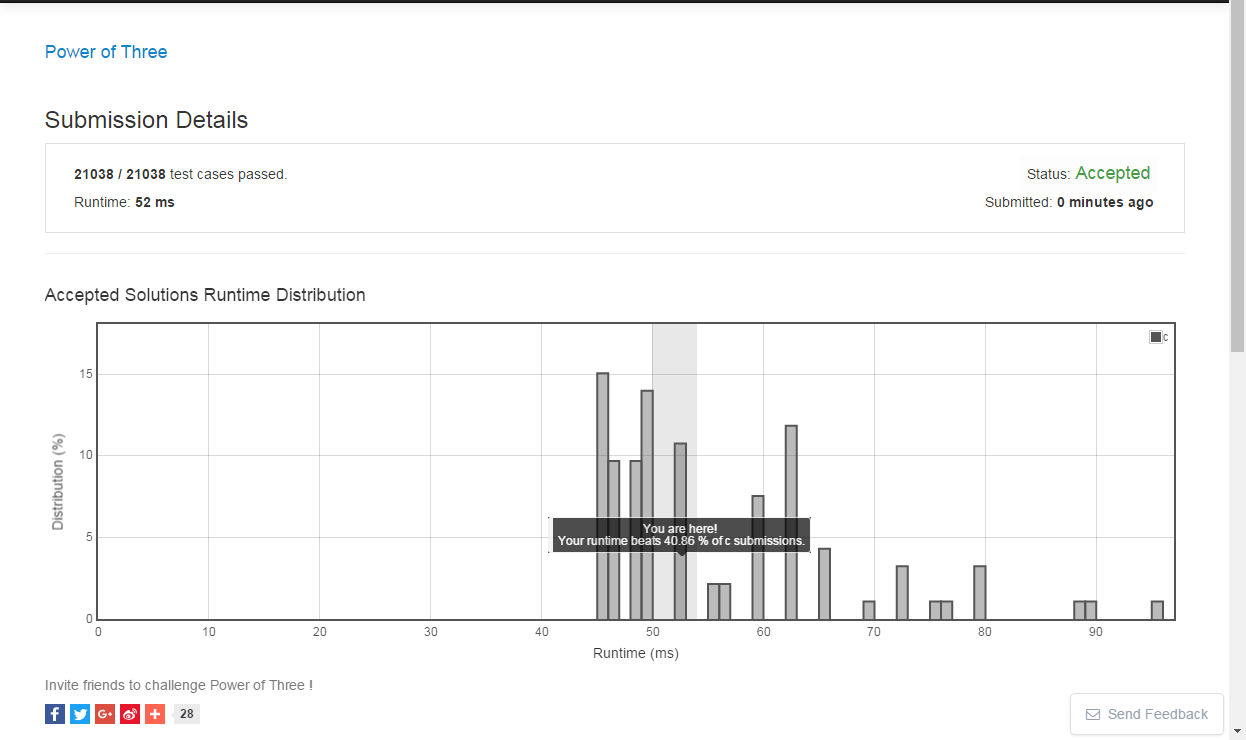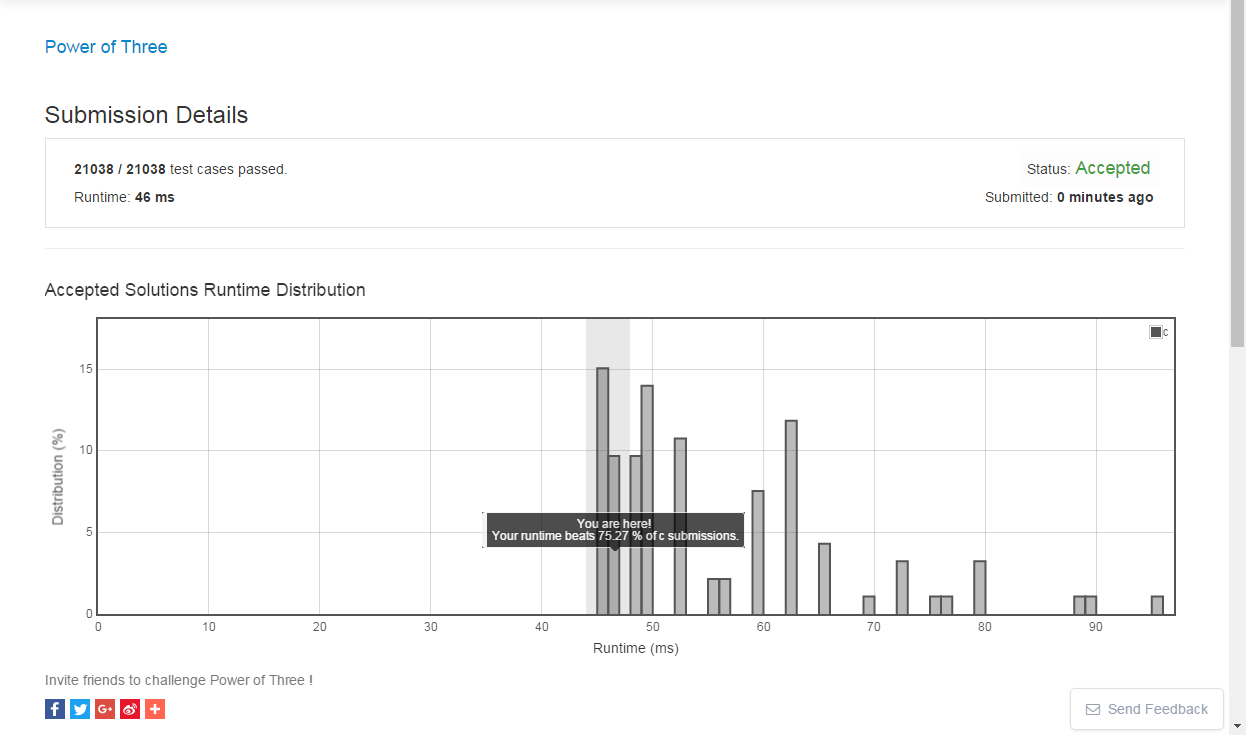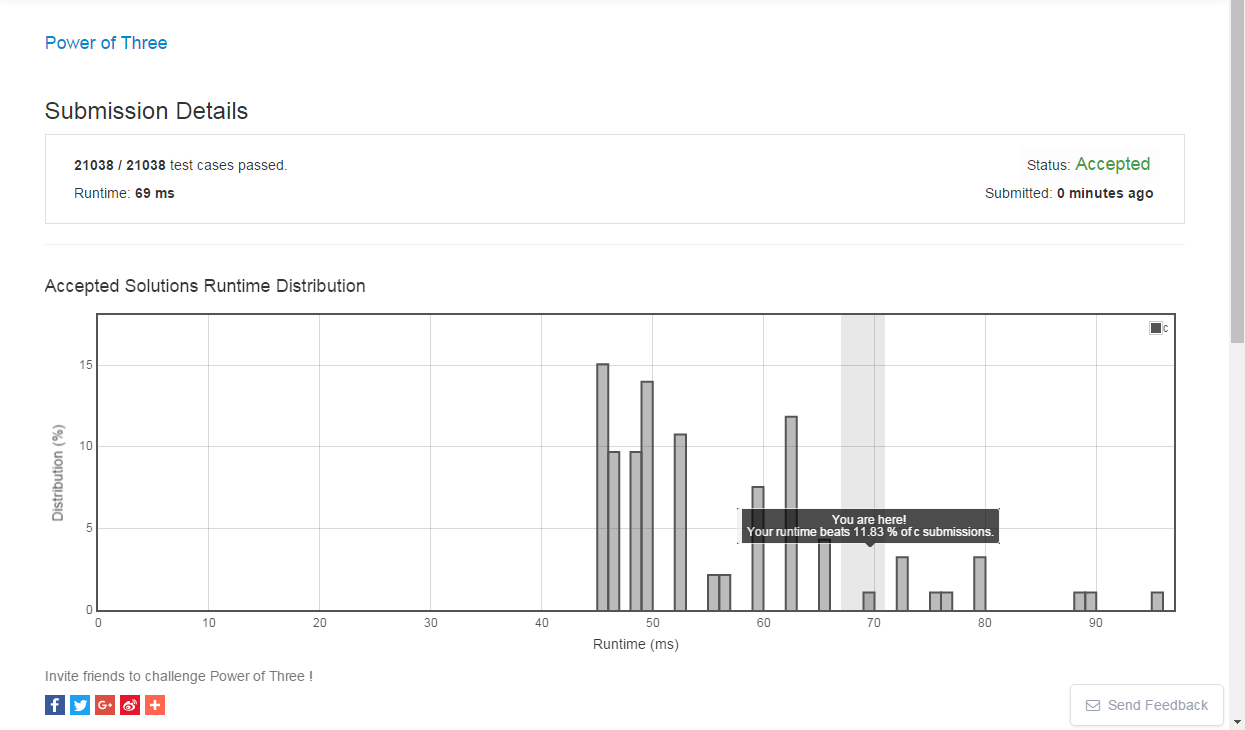# 一、题目

leetcode 上有这么一道题,power of three.

Given an integer, write a function to determine if it is a power of three.

Could you do it without using any loop / recursion?

# 二、解法：

## 1、方法一、

while(n)
{
if(n==1)return true;
if(n%3 != 0)
return false;
n /= 3;
if(n == 1)
return true;
}
return false;

## 2、方法二、

if(n <= 0)return false;
if(1162261467%n == 0)
return true;
else
return false;

## 3、方法三

double res;
res = log10(n)/log10(3);
if(res- (int)res == 0)
return true;
else
return false;

- 1、方法一- 2、方法二- 3、方法三if(n <= 0)return false;
if(4294967296%n == 0)
{
if((int)(log10(n)/log10(2)) % 2 == 0)
return true;
else
return false;
}
else
return false;

# 三、附录

/*
*326. Power of Three
* three ways to solution this problem
*/

#include <stdio.h>
#include <stdbool.h>
#include <math.h>

#define solution 3

bool isPowerOfThree(int n) {

#if solution==1
//循环迭代
while(n)
{
if(n==1)return true;
if(n%3 != 0)
return false;
n /= 3;
if(n == 1)
return true;
}
return false;
#elif solution==2
//32位数中最大的3次方数
if(n <= 0)return false;
if(1162261467%n == 0)
return true;
else
return false;
#elif solution==3
//对数换底公式
//使用 a-(int)a == 0; 来判断a是否为整数
double res;
res = log10(n)/log10(3);
if(res- (int)res == 0)
return true;
else
return false;
#endif
}

int main()
{
int num = 4782968;
bool res = false;

res = isPowerOfThree(num);
printf("res = %d\n",res);

return 0;
}


01-08306
01-1338405-01756
02-24525
05-20185
10-2239
04-2786
12-1737
08-3117
04-03189
07-11410
01-081万+
01-0920
01-052万+
01-111344
01-1821
10-02943
02-06189
02-03157